# 一份简明的 Base64 原理解析

2020/03/07 共 2881 字，约 9 分钟## 1. Base64 是什么

Base64 是一种基于 64 个可打印字符来表示二进制数据的表示方法。由于 2^6=64，所以每 6 个比特为一个单元，对应某个可打印字符。3 个字节有 24 个比特，对应于 4 个 Base64 单元，即 3 个字节可由 4 个可打印字符来表示。

——维基百科

Base64 常用于表示、传输、存储二进制数据，也可以用于将一些含有特殊字符的文本内容编码，以便传输。

1. 在电子邮件的传输中，Base64 可以用来将 binary 的字节序列，比如附件，编码成 ASCII 字节序列；

2. 将一些体积不大的图片 Base64 编码后，直接内嵌到网页源码里；

3. 将要传递给 HTTP 请求的参数做简单的转换，降低肉眼可读性；

注：用于 URL 的 Base64 非标准 Base64，是一种变种。

4. 网友们在论坛等公开场合习惯将邮箱地址 Base64 后再发出来，防止被爬虫抓取后发送垃圾邮件。

## 2. Base64 编码原理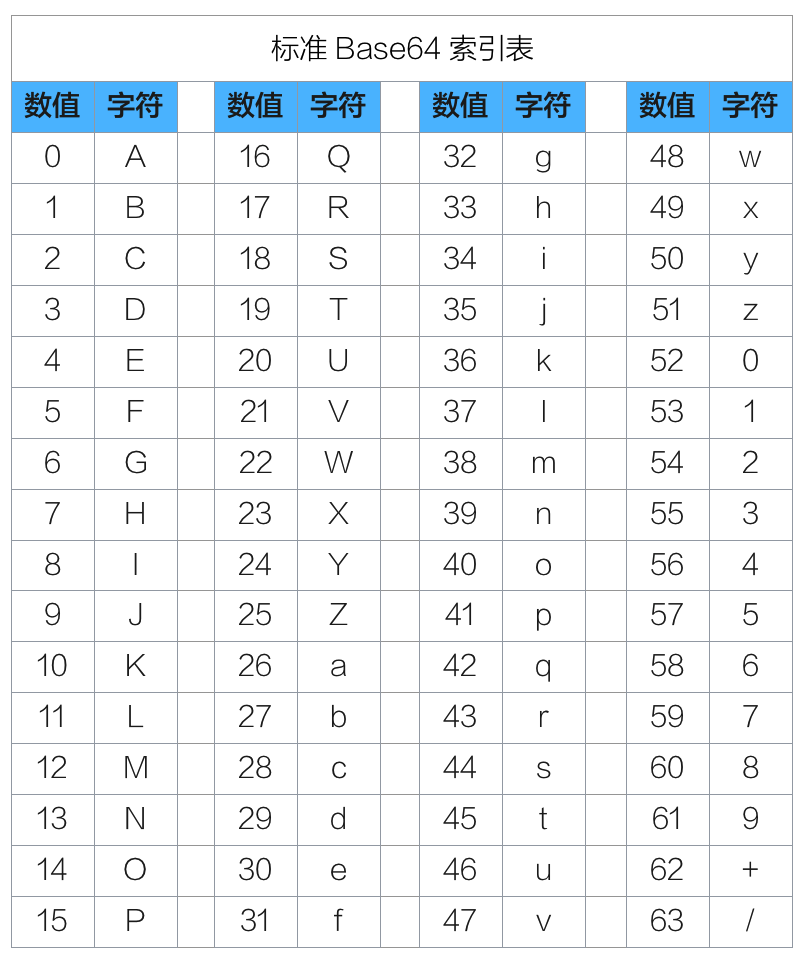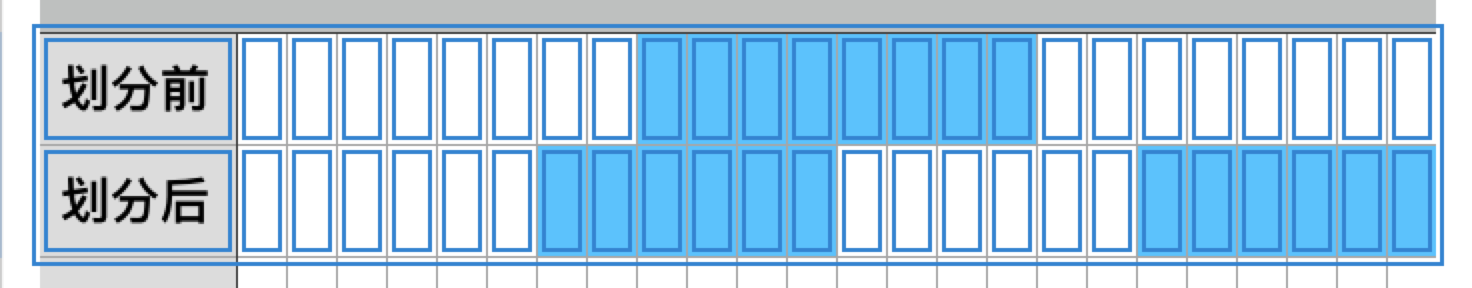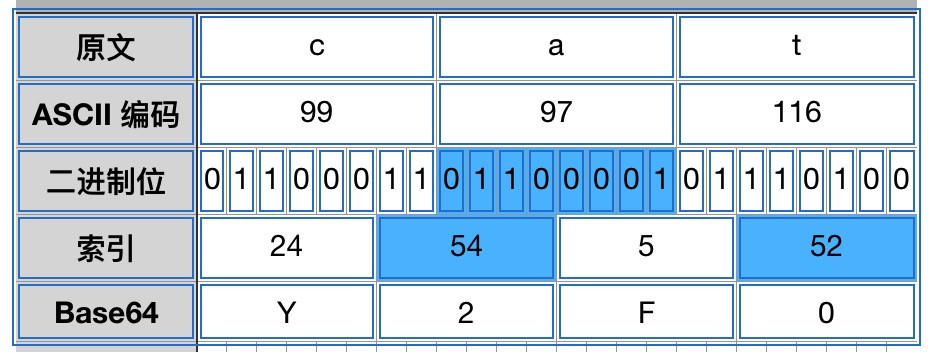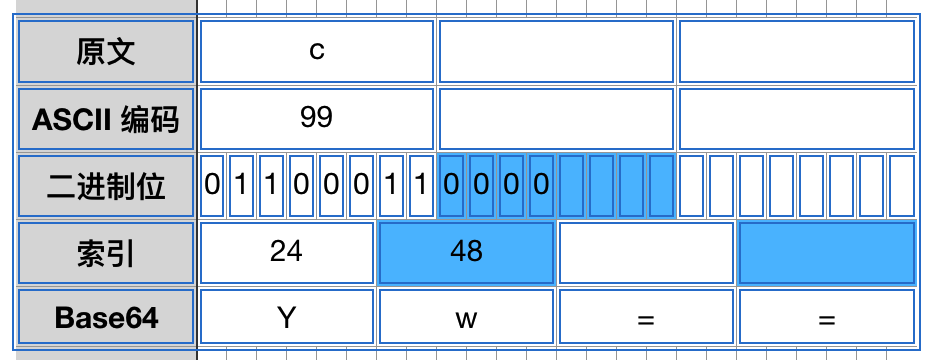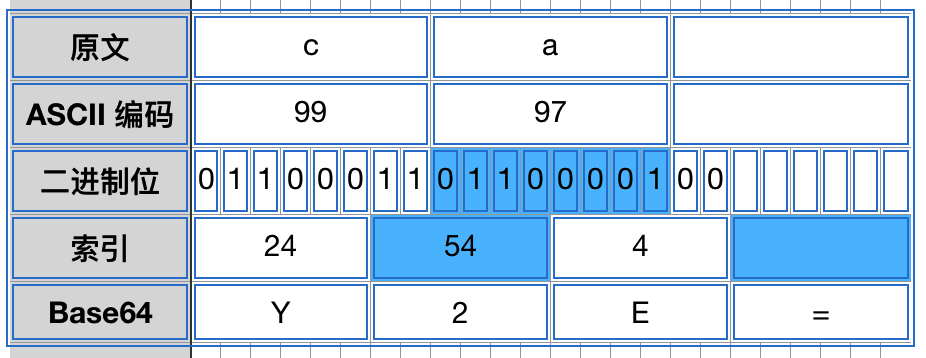## 3. 实现一个简易的 Base64 编码器

``````public class CustomBase64Encoder {

/**
* 索引表
*/
private static final char[] sBase64 = {
'A', 'B', 'C', 'D', 'E', 'F', 'G', 'H',
'I', 'J', 'K', 'L', 'M', 'N', 'O', 'P',
'Q', 'R', 'S', 'T', 'U', 'V', 'W', 'X',
'Y', 'Z', 'a', 'b', 'c', 'd', 'e', 'f',
'g', 'h', 'i', 'j', 'k', 'l', 'm', 'n',
'o', 'p', 'q', 'r', 's', 't', 'u', 'v',
'w', 'x', 'y', 'z', '0', '1', '2', '3',
'4', '5', '6', '7', '8', '9', '+', '/'
};

/**
* 将 byte[] 进行 Base64 编码并返回字符串
* @param src 原文
* @return 编码后的字符串
*/
public static String encode(byte[] src) {
if (src == null) {
return null;
}

byte[] dst = new byte[(src.length + 2) / 3 * 4];

int index = 0;

// 每次将 3 个字节编码为 4 个字节
for (int i = 0; i < (src.length / 3 * 3); i += 3) {
int bits = (src[i] & 0xff) << 16 | (src[i + 1] & 0xff) << 8 | (src[i + 2] & 0xff);
dst[index++] = (byte) sBase64[(bits >>> 18) & 0x3f];
dst[index++] = (byte) sBase64[(bits >>> 12) & 0x3f];
dst[index++] = (byte) sBase64[(bits >>> 6) & 0x3f];
dst[index++] = (byte) sBase64[bits & 0x3f];
}

// 处理剩下的 1 个或 2 个字节
if (src.length % 3 == 1) {
int bits = (src[src.length - 1] & 0xff) << 4;
dst[index++] = (byte) sBase64[(bits >>> 6) & 0x3f];
dst[index++] = (byte) sBase64[bits & 0x3f];
dst[index++] = '=';
dst[index] = '=';
} else if (src.length % 3 == 2) {
int bits = (src[src.length - 2] & 0xff) << 10 | (src[src.length - 1] & 0xff) << 2;
dst[index++] = (byte) sBase64[(bits >>> 12) & 0x3f];
dst[index++] = (byte) sBase64[(bits >>> 6) & 0x3f];
dst[index++] = (byte) sBase64[bits & 0x3f];
dst[index] = '=';
}

return new String(dst);
}
}
``````